# Civil Engineering - Highway Engineering

### Exercise :: Highway Engineering - Section 1

16.

The formula for calculating the depth of concrete pavements suggested by Goldbeck, is

 A.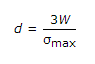B.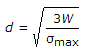C.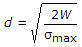D.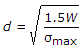Explanation:

No answer description available for this question. Let us discuss.

17.

If A is the projected area of a vehicle in square metres, V is speed of the vehicles in kilometres per hour and C is a constant, then the wind resistance R to the moving vehicles, is given by

 A. R = CAV B. R = CAV2 C. R = CAV3 D.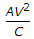E. R = C2AV

Explanation:

No answer description available for this question. Let us discuss.

18.

The usual width of parapet walls along Highways in hilly region, is

 A. 50 cm B. 60 cm C. 70 cm D. 80 cm E. 100 cm

Explanation:

No answer description available for this question. Let us discuss.

19.

The road foundation for modern highways construction, was developed by

 A. Tresguet B. Telford C. Macadam D. Tresguet and Telford simultaneously E. Telford and Macadam simultaneously.

Explanation:

No answer description available for this question. Let us discuss.

20.

If N is deviation angle the length L of a parabolic vetical curve for overtaking sight distance S, is

 A.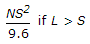B.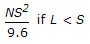C.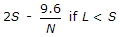D.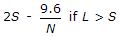E. Both (A) and (C)